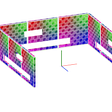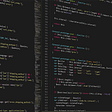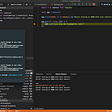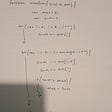# The intersection of Two Lines

The intersection of two lines posted in leetcodes did not seem that complex. Based on the title my initial thought was to find an intersecting x and y-axis, fortunately, I was so wrong. It was similar to the most frequent number problem. The question in Leetcode states “Given two integer arrays nums1 and nums2, return an array of their intersection. Each element in the result must appear as many times as it shows in both arrays and you may return the result in any order.” Here are a few examples below.

`Input: nums1 = [1,2,2,1], nums2 = [2,2]Output: [2,2]Input: nums1 = [4,9,5], nums2 = [9,4,9,8,4]Output: [4,9]Explanation: [9,4] is also accepted.`

Attacking the problem.

I started with sorting the two given arrays. The reasoning behind this was based on the question. I was given the ability to return it in any order. Therefore I decided to sort my numbers and find the element that appears x amount of times. Here’s a closer look at my sorting technique below.

`var intersect = function(nums1, nums2) {        nums1.sort((a,b) => a - b)    nums2.sort((a, b) => a - b)        };`

Right after sorting the given array I created two variables and set them to zero. Both variables would give me the leverage to start at a particular index in the array and fit into my while loop scheme. Furthermore, I created an empty array to return the elements.

`var intersect = function(nums1, nums2) {        nums1.sort((a,b) => a - b)    nums2.sort((a, b) => a - b)        let i = 0    let j = 0    let result = []        };`

I created a while loop that evaluates to true and goes through both arrays. In addition to the loop, I created a conditional statement that compares both arrays to one another. Once that condition is met I continued to increment through the array. Here’s a better look below.

`var intersect = function(nums1, nums2) {        nums1.sort((a,b) => a - b)    nums2.sort((a, b) => a - b)        let i = 0    let j = 0    let result = []        while(i < nums1.length && j < nums2.length){        if(nums1[i] < nums2[j]){            i++        } else if (nums1[i] < nums2[j]){            j++        }     }     };`

At the end of my condition, I added an else statement that grabs the frequent element and pushed it to the first array. Furthermore, I continued to increment through both arrays till all elements were found and returned with my empty array.

`var intersect = function(nums1, nums2) {        nums1.sort((a,b) => a - b)    nums2.sort((a, b) => a - b)        let i = 0    let j = 0    let result = []        while(i < nums1.length && j < nums2.length){        if(nums1[i] < nums2[j]){            i++        } else if (nums1[i] < nums2[j]){            j++        } else {            result.push(nums1[i])             i++            j++        }    }        return result     };`

--

--

--

## More from Woodelin florveus

Love podcasts or audiobooks? Learn on the go with our new app.

## Testing Functional UI the Cloudinary Way## Making holes without a hole punch 🕳## Is it still worth using AngularJS in 2020?## Angular Basics -3## Sending a DELETE Request from a React Application to the Flask API## How to configure live agent functionality using Zendesk?## How to get auto restart and breakpoint support with Typescript and Node## Marek Bálint: I’ve always had support from colleagues, I’ve never been alone for anything